## 5.4.1 The General Framework

The algorithms of Sections 5.4 and 5.5 follow the single-query model, which means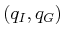is given only once per robot and obstacle set. This means that there are no advantages to precomputation, and the sampling-based motion planning problem can be considered as a kind of search. The multiple-query model, which favors precomputation, is covered in Section 5.6.

The sampling-based planning algorithms presented in the present section are strikingly similar to the family of search algorithms summarized in Section 2.2.4. The main difference lies in step 3 below, in which applying an action,, is replaced by generating a path segment,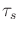. Another difference is that the search graph,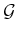, is undirected, with edges that represent paths, as opposed to a directed graph in which edges represent actions. It is possible to make these look similar by defining an action space for motion planning that consists of a collection of paths, but this is avoided here. In the case of motion planning with differential constraints, this will actually be required; see Chapter 14.

Most single-query, sampling-based planning algorithms follow this template:

1. Initialization: Let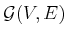represent an undirected search graph, for which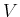contains at least one vertex and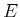contains no edges. Typically,contains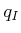,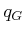, or both. In general, other points inmay be included.
2. Vertex Selection Method (VSM): Choose a vertex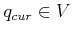for expansion.
3. Local Planning Method (LPM): For some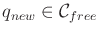that may or may not be represented by a vertex in, attempt to construct a path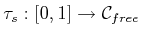such that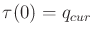and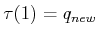. Using the methods of Section 5.3.4,must be checked to ensure that it does not cause a collision. If this step fails to produce a collision-free path segment, then go to step 2.
4. Insert an Edge in the Graph: Insertinto, as an edge from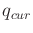to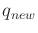. Ifis not already in, then it is inserted.
5. Check for a Solution: Determine whetherencodes a solution path. As in the discrete case, if there is a single search tree, then this is trivial; otherwise, it can become complicated and expensive.
6. Return to Step 2: Iterate unless a solution has been found or some termination condition is satisfied, in which case the algorithm reports failure.

In the present context,is a topological graph, as defined in Example 4.6. Each vertex is a configuration and each edge is a path that connects two configurations. In this chapter, it will be simply referred to as a graph when there is no chance of confusion. Some authors refer to such a graph as a roadmap; however, we reserve the term roadmap for a graph that contains enough paths to make any motion planning query easily solvable. This case is covered in Section 5.6 and throughout Chapter 6.

A large family of sampling-based algorithms can be described by varying the implementations of steps 2 and 3. Implementations of the other steps may also vary, but this is less important and will be described where appropriate. For convenience, step 2 will be called the vertex selection method (VSM) and step 3 will be called the local planning method (LPM). The role of the VSM is similar to that of the priority queue,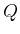, in Section 2.2.1. The role of the LPM is to compute a collision-free path segment that can be added to the graph. It is called local because the path segment is usually simple (e.g., the shortest path) and travels a short distance. It is not global in the sense that the LPM does not try to solve the entire planning problem; it is expected that the LPM may often fail to construct path segments.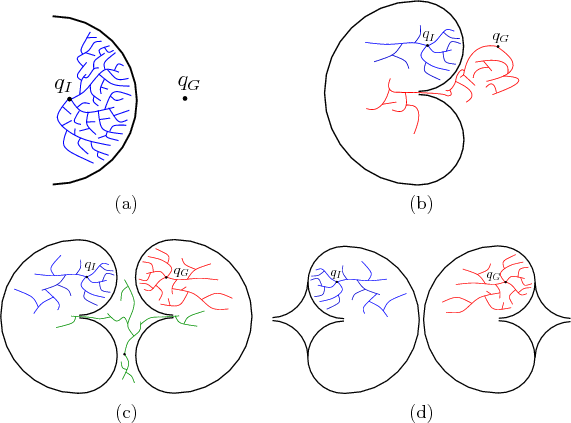It will be formalized shortly, but imagine for the time being that any of the search algorithms from Section 2.2 may be applied to motion planning by approximatingwith a high-resolution grid. The resulting problem looks like a multi-dimensional extension of Example 2.1 (the labyrinth'' walls are formed by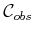). For a high-resolution grid in a high-dimensional space, most classical discrete searching algorithms have trouble getting trapped in a local minimum. There could be an astronomical number of configurations that fall within a concavity inthat must be escaped to solve the problem, as shown in Figure 5.13a. Imagine a problem in which the C-space obstacle is a giant bowl'' that can trap the configuration. This figure is drawn in two dimensions, but imagine that thehas many dimensions, such as six for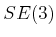or perhaps dozens for a linkage. If the discrete planning algorithms from Section 2.2 are applied to a high-resolution grid approximation of, then they will all waste their time filling up the bowl before being able to escape to. The number of grid points in this bowl would typically be on the order of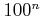for an-dimensional C-space. Therefore, sampling-based motion planning algorithms combine sampling and searching in a way that attempts to overcome this difficulty.

As in the case of discrete search algorithms, there are several classes of algorithms based on the number of search trees.

1. [] Unidirectional (single-tree) methods: In this case, the planning appears very similar to discrete forward search, which was given in Figure 2.4. The main difference between algorithms in this category is how they implement the VSM and LPM. Figure 5.13b shows a bug trap5.9example for which forward-search algorithms would have great trouble; however, the problem might not be difficult for backward search, if the planner incorporates some kind of greedy, best-first behavior. This example, again in high dimensions, can be considered as a kind of bug trap.'' To leave the trap, a path must be found frominto the narrow opening. Imagine a fly buzzing around through the high-dimensional trap. The escape opening might not look too difficult in two dimensions, but if it has a small range with respect to each configuration parameter, it is nearly impossible to find the opening. The tip of the volcano'' would be astronomically small compared to the rest of the bug trap. Examples such as this provide some motivation for bidirectional algorithms. It might be easier for a search tree that starts into arrive in the bug trap.
2. [] Bidirectional (two-tree) methods: Since it is not known whetherormight lie in a bug trap (or another challenging region), a bidirectional approach is often preferable. This follows from an intuition that two propagating wavefronts, one centered onand the other on, will meet after covering less area in comparison to a single wavefront centered atthat must arrive at. A bidirectional search is achieved by defining the VSM to alternate between trees when selecting vertices. The LPM sometimes generates paths that explore new parts of, and at other times it tries to generate a path that connects the two trees.
3. [] Multi-directional (more than two trees) methods: If the problem is so bad that a double bug trap exists, as shown in Figure 5.13c, then it might make sense to grow trees from other places in the hopes that there are better chances to enter the traps in the other direction. This complicates the problem of connecting trees, however. Which pairs of trees should be selected in each iteration for possible connection? How often should the same pair be selected? Which vertex pairs should be selected? Many heuristic parameters may arise in practice to answer these questions.

Of course, one can play the devil's advocate and construct the example in Figure 5.13d, for which virtually all sampling-based planning algorithms are doomed. Even harder versions can be made in which a sequence of several narrow corridors must be located and traversed. We must accept the fact that some problems are hopeless to solve using sampling-based planning methods, unless there is some problem-specific structure that can be additionally exploited.

Steven M LaValle 2020-08-14